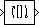Flip

Flip input vertically or horizontally

Library

Signal Management / Indexing

dspindex

•Description

The Flip block vertically or horizontally reverses the M-by-N input matrix, u. The output always has the same dimensionality as the input.

When you set the Flip along parameter to Columns, the block flips the input vertically so the first row of the input becomes the last row of the output.

y = flipud(u)						% Equivalent MATLAB code

When flipping the input vertically, the block treats length-M unoriented vector inputs as M-by-1 column vectors.

When you set the Flip along parameter to Rows, the block flips the input horizontally so the first column of the input becomes the last column of the output.

y = fliplr(u)						% Equivalent MATLAB code

When flipping the input horizontally, the block treats length-N unoriented vector inputs as 1-by-N row vectors.

This block supports Simulink® virtual buses.

Parameters

Flip along

Specify the dimension along which to flip the input. When you set this parameter to Columns, the block flips the input vertically. When you set this parameter to Rows, the block flips the input horizontally.

Supported Data Types

PortSupported Data Types

Input

• Double-precision floating point

• Single-precision floating point

• Fixed point (signed and unsigned)

• Boolean

• 8-, 16-, and 32-bit signed integers

• 8-, 16-, and 32-bit unsigned integers

• Enumerated

Output

• Double-precision floating point

• Single-precision floating point

• Fixed point (signed and unsigned)

• Boolean

• 8-, 16-, and 32-bit signed integers

• 8-, 16-, and 32-bit unsigned integers

• Enumerated

 Selector (Simulink) Simulink Variable Selector DSP System Toolbox flipud MATLAB fliplr MATLAB

Extended Capabilities

Fixed-Point ConversionDesign and simulate fixed-point systems using Fixed-Point Designer™.

Introduced before R2006a

DSP System Toolbox DocumentationGet trial now